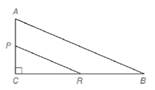Chapter 5.2, Problem 10E### Elementary Geometry for College St...

6th Edition
Daniel C. Alexander + 1 other
ISBN: 9781285195698

#### Solutions

Chapter
Section### Elementary Geometry for College St...

6th Edition
Daniel C. Alexander + 1 other
ISBN: 9781285195698
Textbook Problem
4 views

# Given: △ A B C ∼ △ P R C , m ∠ A = 67 ∘ , P C = 5 , C R = 12 , P R = 13 , A B = 26 Find: a) m ∠ B b) m ∠ R P C c) A C d) C BTo determine

a)

To calculate:

Find the angle mB.

Explanation

Given:

ΔABC~ΔPRCmA=67PC=5,CR=12,PR=13,and AB=26

Definition Used:

Sum of all the angles in a triangle is 180.

Calculate:

Here, mC=90

To determine

b)

To calculate:

Find the angle RPC.

To determine

c)

To calculate:

The value of AC.

To determine

d)

To calculate:

The value of CB.

### Still sussing out bartleby?

Check out a sample textbook solution.

See a sample solution

#### The Solution to Your Study Problems

Bartleby provides explanations to thousands of textbook problems written by our experts, many with advanced degrees!

Get Started

#### Find the derivatives of the functions in Problems 1-34. 19.

Mathematical Applications for the Management, Life, and Social Sciences

#### In Exercises 5-8, graph the given function or equation. 2x3y=12

Finite Mathematics and Applied Calculus (MindTap Course List)

#### let f(x) = x3 + 5, g(x) = x2 2, and h(x)= 2x + 4. Find the rule for each function. 3. fg

Applied Calculus for the Managerial, Life, and Social Sciences: A Brief Approach

#### What is the value of (X + 1) for the following scores: 0, 1, 4, 2? a. 8 b. 9 c. 11 d. 16

Essentials of Statistics for The Behavioral Sciences (MindTap Course List)

#### an=5n

Calculus: Early Transcendental Functions (MindTap Course List)